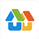# 北师大版三年上册数学6.5

2019-06-02 09:42:00    说两句一个乘数中间或末尾有0的乘法

1.口算

0×6=9+0=72×0=0×9=

0+732=104－0=4×0=0+13=

0×0=100×0=0×245=90+0=

2.竖式计算

370×2=140×2=308×5=

3.滨湖家园新建了8幢居民楼，每幢住104户。新建的楼一共能住多少户？

4.王老师打一篇1000字的文章，如果每分钟打109个字，他9分钟能打完吗？

欢迎访问奥数网，您还可以通过手机等移动设备查询小学试题库、小学资源库、小升初动态、家庭教育、重点中学信息等，2020小升初我们一路相伴。>>[点击查看]

•欢迎扫描二维码
关注奥数网微信
ID：aoshu_2003

•欢迎扫描二维码
关注中考网微信
ID：zhongkao_com

## 奥数关键词